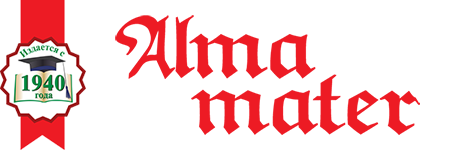ISSN 1026-955X
Vestnik Vysshey Shkoly (Higher School Herald)
The best way to learn all about Higher Education
Languages

=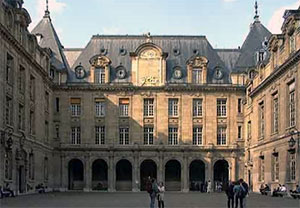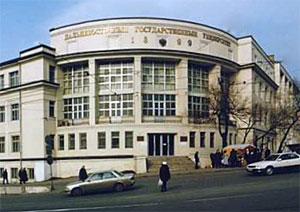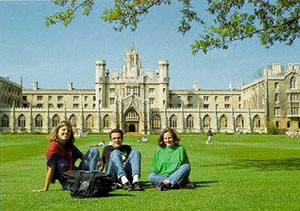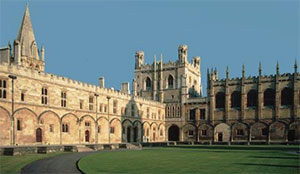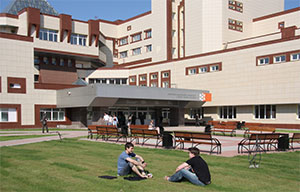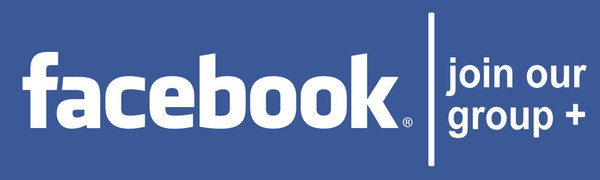# Methodological unity of mathematic in the context of philosophy of education

N.V. Mikhailova
80,00 Р

N.V. Mikhailova is Cand. Sci. (Philosophy), doc. at Belorussian State University of Informatics & Radio-Electronics e-mail: michailova_mshrc@mail.ru

Elaborated is the theme of methodological unity of mathematic in the context of philosophy of education. Presented is argumentation of methodological unity of contemporary mathematics, which potentially contributes to understanding of problems of philosophy of mathematical education. Discussed are such aspects of examining theme, as philosophy of mathematical education, structural unity of mathematic, pure and applied mathematics, the role of mathematic as language of science, etc. Also used are statements of professional mathematicians on examining topic, and presented is detailed analysis of various points of view on this problem. The author’s conclusion is made, that internal evolution of mathematical science more than ever strengthened unity of ways of it’s development, which should be reflected in mathematical education.

Key words: philosophy of mathematical education, unity of mathematics, teaching in mathematics.

References

1. Atiyah, M. Mathematics in the twentieth century. Mathematical education. Ser. 3, 2003, Iss. 7, pp. 5–24.

2. Perminov, V.Ya. Round table “Mathematics and reality”. Bulletin of Vyatka state humanities university. 2011, no. 1, pp. 16–20.

3. Kolmogorov, A.N. Mathematics in it’s historical development. Moscow, 2007.

4. Stuart, J. Mathematics of 2050 year. In: The future of science in the XXI century. Following fifty years. Moscow, 2011, pp. 37–46.

5. Courant, R. Mathematics in the contemporary world. Moscow, 1967, pp. 13–27.

6. Arnold, V.I. Mathematics with a human face. Nature, 1988, no. 3, pp. 117–119.

7. Smirnov, S. About modern mathematics and it’s teaching. Quantum. 2011, no. 2, pp. 11–18.

8. Poincaré, A. Logic and intuition in mathematical science and teaching. In: Recent works. Izhevsk, 2001, pp. 19–24.

9. Matiyasevich, Yu.V. Mathematical proof: yesterday, today, tomorrow. Computer tools in education, 2012, no. 6, pp. 13–24.